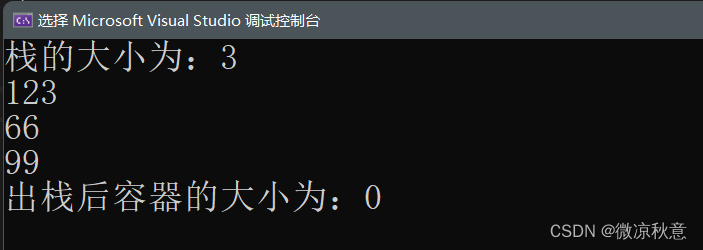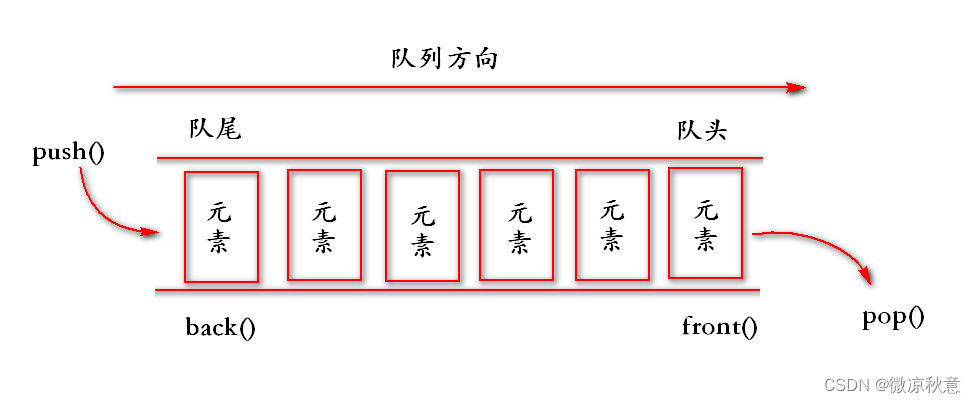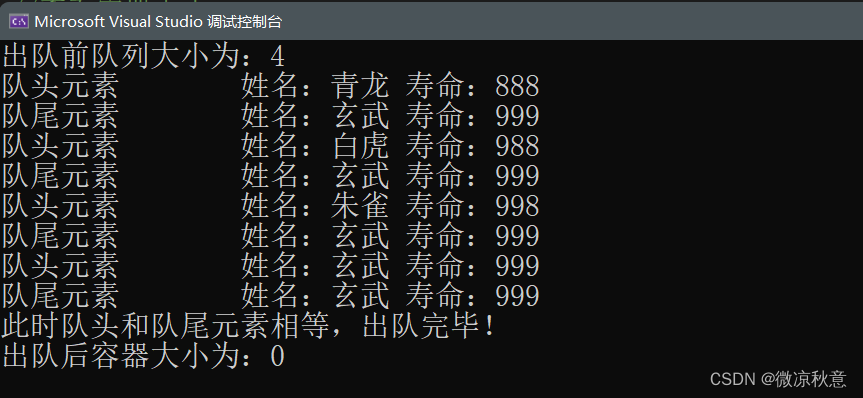> 文档中心 > ＜C++＞ stack与queue容器概念模型|常用接口汇总

# ＜C++＞ stack与queue容器概念模型|常用接口汇总

✅作者简介：热爱后端语言的大学生，CSDN内容合伙人
✨精品专栏：C++面向对象
🔥系列专栏：C++泛型编程

### 文章目录

• 1、stack 容器概念模型
• 1.1、stack 容器模型图
• 1.2、stack 基本概念与特点
• 2、stack 容器常用接口
• 2.1、stack 代码综合使用
• 3、queue 容器概念模型
• 3.1、queue 容器模型图
• 3.2、queue 基本概念与特点
• 4、queue 容器常用接口
• 4.1、queue 代码综合使用

🔥前言

# 1、stack 容器概念模型

## 1.1、stack 容器模型图## 1.2、stack 基本概念与特点

stack栈(堆栈)，是一种先进后出(`First In Last Out,FILO`)的数据结构，其中一端封闭。

• 只有位于栈顶元素才可以被外界访问
• 栈用进入数据称为入栈：`push`
• 栈中弹出数据成为出栈：`pop`
• stack 不支持遍历操作
• 遍历需要在不改变原存储空间的条件下进行
• 通过每次出栈来访问所有元素的方式不能叫遍历
• 能够判断容器是否为空和得到元素个数
• 通过入栈来计数，并不是对出栈计数

# 2、stack 容器常用接口

• `stackstk;`
• 默认构造，`T` 采用了模板类的写法，存放数据类型
• `stack(const stack &stk);`
• 拷贝构造函数

• `stack& operator=(const stack &stk);`
• 重载赋值运算符，可直接进行赋值

• `push(elem);`
• 入栈操作，向栈顶添加元素
• `pop();`
• 出栈操作，从栈顶移除元素
• `top();`
• 返回栈顶元素

• `empty();`
• 判断堆栈是否为空
• `size();`
• 返回栈的大小

## 2.1、stack 代码综合使用

``#include#includeusing namespace std;int main(){//默认构造创建栈stack<int>stk;//添加元素stk.push(99);stk.push(66);stk.push(123);//查看此时容器大小cout <<"栈的大小为："<< stk.size() << endl;//结合接口来查看栈内元素while (!stk.empty()){//访问栈顶元素cout << stk.top() << endl;//出栈，用于更新栈顶元素stk.pop();}//查看此时容器大小cout << "出栈后容器的大小为：" << stk.size() << endl;return 0;}``# 3、queue 容器概念模型

## 3.1、queue 容器模型图## 3.2、queue 基本概念与特点

queue队列，是一种先进先出(`First In First Out,FIFO`)的数据结构，它有两个出口。

• 允许从一端添加元素，另一端删除元素
• 只有队头和队尾可以被外界访问，因此不支持遍历操作
• 入队操作：`push`，出队操作：`pop`
• 能够判断容器是否为空和得到元素个数

# 4、queue 容器常用接口

`queue``stack`常用接口几乎一致，唯一不同的就是队列的队首和队尾与堆栈的栈顶表示有所不同。

• `front()`接口用来返回队首元素
• `back()` 接口用来返回队尾元素

## 4.1、queue 代码综合使用

``//定义队列，含重载相等运算符class Student{public:Student(string name, int age){this->name = name;this->age = age;}//重载相等关系运算符bool operator==(Student& s){if (s.name == this->name) {if (s.age = this->age)return true;elsereturn false;}else return false;}string name;int age;};void testQueue(){//创建空队列queue<Student>Q;//准备Student数据Student s1("青龙", 888);Student s2("白虎", 988);Student s3("朱雀", 998);Student s4("玄武", 999);//入队Q.push(s1);Q.push(s2);Q.push(s3);Q.push(s4);//查看容器大小cout << "出队前队列大小为：" << Q.size() << endl;//查看队头队尾元素、出队操作while (!Q.empty()){cout << "队头元素\t姓名：" << Q.front().name << " 寿命：" << Q.front().age << endl;cout << "队尾元素\t姓名：" << Q.back().name << " 寿命：" << Q.back().age << endl;if (Q.front() == Q.back()) {cout << "此时队头和队尾元素相等，出队完毕！" << endl;}Q.pop();}//查看容器大小cout << "出队后容器大小为：" << Q.size() << endl;}``